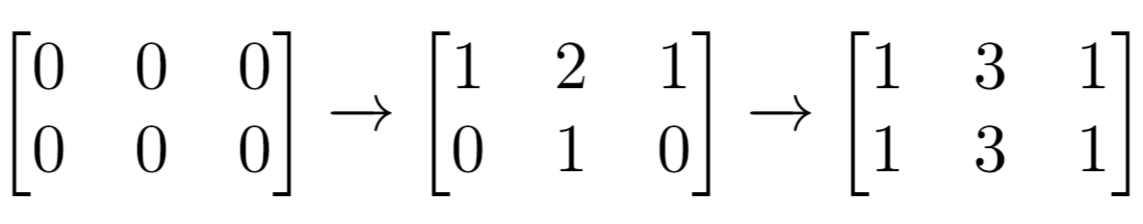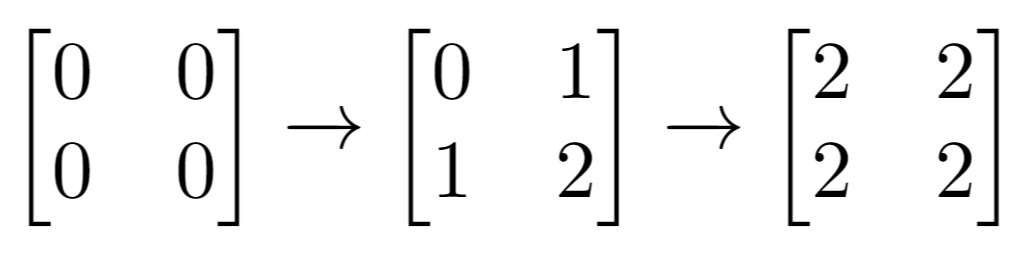# LeetCode: 1252. 奇数值单元格的数目¶

## 1、题目描述¶输入：n = 2, m = 3, indices = [[0,1],[1,1]]输入：n = 2, m = 2, indices = [[1,1],[0,0]]



• $1 <= n <= 50$
• $1 <= m <= 50$
• $1 <= indices.length <= 100$
• $0 <= indices[i] < n$
• $0 <= indices[i] < m$

## 2、解题思路¶

• 针对每一个点，能够增加1的情况就是行和列包括这个点的横纵坐标，只需要统计出这个点横纵坐标被包含了多少次就得到最终的值
from collections import defaultdict

class Solution:
def oddCells(self, n: int, m: int, indices: List[List[int]]) -> int:
ans = 0

rows = defaultdict(int)
cols = defaultdict(int)
for x, y in indices:
rows[x] += 1
cols[y] += 1
for i in range(n):
for j in range(m):
if rows[i] + cols[j] & 1:
ans += 1
return ans